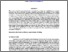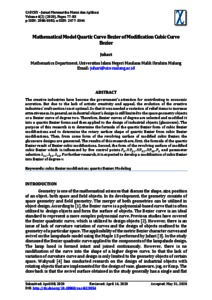# Mathematical model quartic curve Bezier of modification cubic curve Bezier

Juhari, Juhari (2020) Mathematical model quartic curve Bezier of modification cubic curve Bezier. Cauchy: Jurnal Matematika Murni dan Aplikasi, 6 (2). pp. 77-83. ISSN 2086-0382Preview
Text
4. juhari (77-83).pdf - Published Version

Full text available at: http://ejournal.uin-malang.ac.id/index.php/Math/ar...

## Abstract

The creative industries have become the government's attention for contributing to economic accretion. But due to the lack of artistic creativity and appeal, the evolution of the creative industries' craft section is not optimal. So that it was needed a variation of relief items to increase attractiveness. In general, an industrial object's design is still limited to the space geometry objects or a Bezier curve of degree two. Therefore, Bezier curves of degree are selected and modified it into a quartic Bezier forms and then applied to the design of industrial objects (glassware). The purpose of this research is to determine the formula of the quartic Bezier form of cubic Bezier modifications and to determine the rotary surface shape of quartic Bezier from cubic Bezier modifications. Then, from some form of the revolving surface of modified cubic Bezier, the glassware designs are generated. The results of this research are, first, the formula of the quartic Bezier result of Bezier cubic modifications. Second, the form of the revolving surface of modified cubic Bezier which is influenced by five control points P_0,NP_31,NP_32,NP_33,P_3 and parameter selection λ_31,λ_32,λ_33. For further research, it is expected to develop a modification of cubic Bezier into Bezier of degree-n

Item Type: Journal Article Cubic Bezier modification; quartic Bezier; Modeling 01 MATHEMATICAL SCIENCES > 0101 Pure Mathematics > 010102 Algebraic and Differential Geometry01 MATHEMATICAL SCIENCES > 0101 Pure Mathematics > 010199 Pure Mathematics not elsewhere classified Faculty of Mathematics and Sciences > Department of Mathematics Juhari Juhari 06 May 2020 09:26View Item